International
Tables for
Crystallography
Volume F
Crystallography of biological macromolecules
Edited by M. G. Rossmann and E. Arnold

International Tables for Crystallography (2006). Vol. F, ch. 15.2, pp. 325-327   | 1 | 2 |

Section 15.2.3. Structure-factor probability relationships

aDepartment of Haematology, University of Cambridge, Wellcome Trust Centre for Molecular Mechanisms in Disease, CIMR, Wellcome Trust/MRC Building, Hills Road, Cambridge CB2 2XY, England
Correspondence e-mail: rjr27@cam.ac.uk

15.2.3. Structure-factor probability relationships

| top | pdf |

To use model phase information optimally, the probability distribution for the true phase (or, equivalently, the distribution of the error in the model phase) needs to be known. Such a distribution can be derived by first working out the probability distribution for the true structure factor (or the distribution of the vector difference between the model and true structure factors). Then the phase probability distribution is obtained by fixing the known value of the structure-factor amplitude and renormalizing.

A number of related structure-factor distributions have been derived, differing in the amount of information available about the structure and in the assumed form of errors in the model. These range from the Wilson distribution, which applies when none of the atomic positions is known, to a distribution that applies when there are a variety of sources of error in an atomic model.

15.2.3.1. Wilson and Sim structure-factor distributions in P1

| top | pdf |

For the Wilson distribution (Wilson, 1949), it is assumed that the atoms in a crystal structure in space group P1 are scattered randomly and independently through the unit cell. In fact, it is sufficient to make the much less restrictive assumption that the atoms are placed randomly with respect to the Bragg planes defined by the Miller indices. The assumption of independence is somewhat more problematic, since there are restrictions on the distances between atoms, large volumes of protein crystals are occupied by disordered solvent and many protein crystals display noncrystallographic symmetry; as discussed elsewhere (Vellieux & Read, 1997), the resulting relationships among structure factors are exploited implicitly in averaging and solvent-flattening procedures. The higher-order relationships among structure factors are used explicitly in direct methods for solving small-molecule structures and are being developed for use in protein structures (Bricogne, 1993). For the purposes of simpler relationships between the calculated and true structure factors for a single hkl, however, the lack of complete independence does not seem to create serious problems.

When atoms are placed randomly relative to the Bragg planes, the contribution of each atom to the structure factor will have a phase varying randomly from 0 to 2π. The overall structure factor can then be considered to be the result of a random walk in the complex plane, which can be treated as an application of the central limit theorem. The structure factor is the sum of the independent atomic scattering contributions, each of which has a probability distribution defined as a circle in the complex plane centred on the origin, with a radius of. The centroid of this atomic distribution is at the origin, and the variance for each of the real and imaginary parts is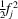. The probability distribution of the structure factor that is the sum of these contributions is a two-dimensional Gaussian, the product of the one-dimensional Gaussians for the real and imaginary parts. Because the variances are equal in the real and imaginary directions, it can be simplified, as shown below, and expressed in terms of a single distribution parameter,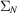.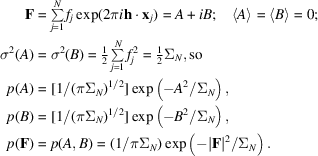The Sim distribution (Sim, 1959), which is relevant when the positions of some of the atoms are known, has a very similar basis, except that the structure factor is now considered to arise from a random walk starting from the position of the structure factor corresponding to the known part,. Atoms with known positions do not contribute to the variance, while each of the atoms with unknown positions (the Q' atoms) contributesto each of the real and imaginary parts, as in the Wilson distribution. The distribution parameter in this case is referred to as. The Sim distribution is a conditional probability distribution, depending on the value of,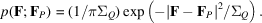The Wilson (1949)and Woolfson (1956)distributions for space groupare obtained similarly, except that the random walks are along a line and the resulting Gaussian distributions are one-dimensional. (The Woolfson distribution is the centric equivalent of the Sim distribution.) For more complicated space groups, it is reasonable to assume that acentric reflections follow the P1 distribution and that centric reflections follow thedistribution. However, for any zone of the reciprocal lattice in which symmetry-related atoms are constrained to scatter in phase, the variances must be multiplied by the expected intensity factor,, for the zone, because the symmetry-related contributions are no longer independent.

15.2.3.2. Probability distributions for variable coordinate errors

| top | pdf |

In the Sim distribution, an atom is considered to be either exactly known or completely unknown in its position. These are extreme cases, since there will normally be varying degrees of uncertainty in the positions of various atoms in a model. The treatment can be generalized by allowing a probability distribution of coordinate errors for each atom. In this case, the centroid for the individual atomic contribution to the structure factor will no longer be obtained by multiplying by either zero or one. Averaged over the circle corresponding to possible phase errors, the centroid will generally be reduced in magnitude, as illustrated in Fig. 15.2.3.1.In fact, averaging to obtain the centroid is equivalent to weighting the atomic scattering contribution by the Fourier transform of the coordinate-error probability distribution,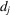. By the convolution theorem, this in turn is equivalent to convoluting the atomic density with the coordinate-error distribution. Intuitively, the atom is smeared over all of its possible positions. The weighting factor,, is thus analogous to the thermal-motion term in the structure-factor expression.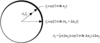Figure 15.2.3.1 | top | pdf |Centroid of the structure-factor contribution from a single atom. The probability of a phase for the contribution is indicated by the thickness of the line.

The variances for the individual atomic contributions will differ in magnitude, but if there are a sufficient number of independent sources of error, we can invoke the central limit theorem again and assume that the probability distribution for the structure factor will be a Gaussian centred on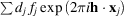. If the coordinate-error distribution is Gaussian, and if each atom in the model is subject to the same errors, the resulting structure-factor probability distribution is the Luzzati (1952)distribution. In this special case,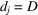for all atoms, where D is the Fourier transform of a Gaussian and behaves like the application of an overall B factor.

15.2.3.3. General treatment of the structure-factor distribution

| top | pdf |

The Wilson, Sim, Luzzati and variable-error distributions have very similar forms, because they are all Gaussians arising from the application of the central limit theorem. The central limit theorem is valid under many circumstances; even when there are errors in position, scattering factor and B factor, as well as missing atoms, a similar distribution still applies. As long as these sources of error are independent, the true structure factor will have a Gaussian distribution centred on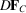(Fig. 15.2.3.2),where D now includes effects of all sources of error, as well as compensating for errors in the overall scale and B factor (Read, 1990).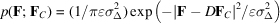in the acentric case, where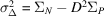,is the expected intensity factor and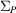is the Wilson distribution parameter for the model.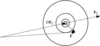Figure 15.2.3.2 | top | pdf |Schematic illustration of the general structure-factor distribution, relevant in the case of any set of independent random errors in the atomic model.

For centric reflections, the scattering differences are distributed along a line, so the probability distribution is a one-dimensional Gaussian.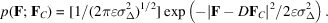15.2.3.4. Estimating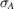| top | pdf |

Srinivasan (1966)showed that the Sim and Luzzati distributions could be combined into a single distribution that had a particularly elegant form when expressed in terms of normalized structure factors, or E values. This functional form still applies to the general distribution that reflects a variety of sources of error; the only difference is the interpretation placed on the parameters (Read, 1990). If F and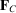are replaced by the corresponding E values, a parameterplays the role of D, and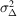reduces to (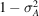). [The parameteris equivalent to D after correction for model completeness;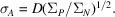] When the structure factors are normalized, overall scale and B-factor effects are also eliminated. The parameterthat characterizes this probability distribution varies as a function of resolution. It must be deduced from the amplitudes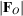and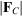, since the phase (thus the phase difference) is unknown.

A general approach to estimating parameters for probability distributions is to maximize a likelihood function. The likelihood function is the overall joint probability of making the entire set of observations, which is a function of the desired parameters. The parameters that maximize the probability of making the set of observations are the most consistent with the data. The idea of using maximum likelihood to estimate model phase errors was introduced by Lunin & Urzhumtsev (1984), who gave a treatment that was valid for space group P1. In a more general treatment that applies to higher-symmetry space groups, allowance is made for the statistical effects of crystal symmetry (centric zones and differing expected intensity factors) (Read, 1986).

Thevalues are estimated by maximizing the joint probability of making the set of observations of. If the structure factors are all assumed to be independent, the joint probability distribution is the product of all the individual distributions. The assumption of independence is not completely justified in theory, but the results are fairly accurate in practice.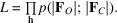The required probability distribution,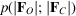, is derived from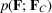by integrating over all possible phase differences and neglecting the errors inas a measure of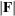. The form of this distribution, which is given in other publications (Read, 1986, 1990), differs for centric and acentric reflections. (It is important to note that although the distributions for structure factors are Gaussian, the distributions for amplitudes obtained by integrating out the phase are not.) It is more convenient to deal with a sum than a product, so the log likelihood function is maximized instead. In the program SIGMAA, reciprocal space is divided into spherical shells, and a value of the parameteris refined for each resolution shell. Details of the algorithm are given elsewhere (Read, 1986).

The resolution shells must be thick enough to contain several hundred to a thousand reflections each, in order to provideestimates with a sufficiently small statistical error. A larger number of shells (fewer reflections per shell) can be used for refined structures, since estimates ofbecome more precise as the true value approaches 1. If there are sufficient reflections per shell, the estimates will vary smoothly with resolution. As discussed below, the smooth variation with resolution can also be exploited through a restraint that allowsvalues to be estimated from fewer reflections.

References

Bricogne, G. (1993). Direct phase determination by entropy maximization and likelihood ranking: status report and perspectives. Acta Cryst. D49, 37–60.Google Scholar
Lunin, V. Yu. & Urzhumtsev, A. G. (1984). Improvement of protein phases by coarse model modification. Acta Cryst. A40, 269–277.Google Scholar
Luzzati, V. (1952). Traitement statistique des erreurs dans la determination des structures cristallines. Acta Cryst. 5, 802–810.Google Scholar
Read, R. J. (1986). Improved Fourier coefficients for maps using phases from partial structures with errors. Acta Cryst. A42, 140–149.Google Scholar
Read, R. J. (1990). Structure-factor probabilities for related structures. Acta Cryst. A46, 900–912.Google Scholar
Sim, G. A. (1959). The distribution of phase angles for structures containing heavy atoms. II. A modification of the normal heavy-atom method for non-centrosymmetrical structures. Acta Cryst. 12, 813–815.Google Scholar
Srinivasan, R. (1966). Weighting functions for use in the early stages of structure analysis when a part of the structure is known. Acta Cryst. 20, 143–144.Google Scholar
Vellieux, F. M. D. & Read, R. J. (1997). Non-crystallographic symmetry averaging in phase refinement and extension. Methods Enzymol. 277, 18–53.Google Scholar
Wilson, A. J. C. (1949). The probability distribution of X-ray intensities. Acta Cryst. 2, 318–321.Google Scholar
Woolfson, M. M. (1956). An improvement of the heavy-atom' method of solving crystal structures. Acta Cryst. 9, 804–810.Google Scholar